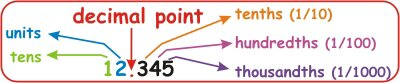# 4th Grade Math Learning - Math Worksheets and Activities - MyHomeSchoolMath

myhomeschoolmath.comTitle
Go to content## 4th Grade Math Learning - Math Worksheets and Activities

Free printable Math Worksheets - 4th grade
This is a collection of math worksheets for grade 4, organized by topics such as comparing, rounding, place value, addition, subtraction, mental math, multiplication, division, equations, inverse operations, fractions, decimals, measuring and geometry.
The worksheets are, except in pdf form, randomly generated each time you click on the links below. You can also get a new, different one just by refreshing the page in your browser.
Numbers, Comparing, Place value